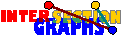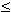# Distance

Topological properties of the representation may be reflected in the intersection graph. Even metric properties, like distance, sometimes show through.

Why is every power of an interval graphs an interval graphs, but not every power of a unit interval graph a unit interval graph? Why are odd powers of chordal graphs chordal, but the even powers not necessarily?

Let G be the intersection graph of the hypergraphs H=(A,(Sx/xV)). Then distances in G can be expressed by set intersection of the sets Sxt, where Sx1 :=Sx and Sxt is recursively defined. We get dG(x,y)i+j-1 if and only if SxiSyj is nonempty. Therefore (A,(Sxt/xV)) is a intersection representation of the (2t-1)th power of G, whose vertices are adjacent if and only if dG(x,y)2t. In some situations, these sets Sxt have the same shape than the original sets Sx. For instance, if the only property P the sets Sx have to be obey is, that they should be connected subsets of some topological space, then the sets Sxt are also connected subsets of it. Then every odd power of a graph in G(P) lies again in G(P). A prominent example is the classe of chordal graphs[BP83], [D84]: Odd powers of chordal graphs are chordal.

But we get an odd power result also for circular-arc graphs or interval graphs in this way.

What about even powers? Here we need geometric models with ordering. Assume G is the intersection graph of P-sets Sx, where for every two disjoint sets, one is to the left of the other. Assume furthermore that Sy \ Sx divides naturally into two parts, one to the left and the other to the right of Sx. Then we may define Rx as the union of Sx and all right parts of Sy \ Sx for neighbors y of x. It turns out that these sets Rx are an intersection representation of G2. The only problem may be that these sets are not necessarily P-sets. In some cases, however, we may replace them by P-sets having the same intersection pattern than the sets Rx.

If the Sxt-construction above applies also, we get representations of all powers of G by P-sets in that case.

This approach works for intervals on the real line without further ado. It works for trapezoids between two parallel lines by taking the convex hulls of the sets Rx constructed (which are no trapezoids). It even works for structures with some cyclic ordering, as for connected subsets (circular arcs) of the unit circle.[R87] All powers of interval graphs are interval graphs. [F95] All powers of trapezoid graphs are trapezoid graphs. [D92] All powers of cocomparability graphs are cocomparability graphs. [R92] All powers of circular-arc graphs are circular-arc graphs.A. Raychaudhouri posed the problem whether or not it is true that whenever Gk is a circular-arc graph, then Gk+1 must too be a circular-arc graph.

There are several other situations where the distances transfer, let me just mention one more example for line graphs and clique graphs:[H84], [H86]: For every graph G, diam(G)-1diam(L(G)diam(G)+1, as well as diam(G)-1diam(C(G)diam(G)+1.

Back to the start page for intersection graphs.

Erich Prisner